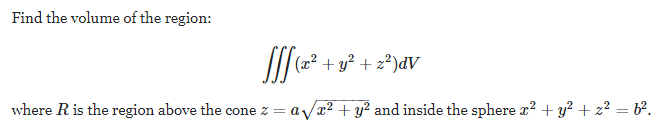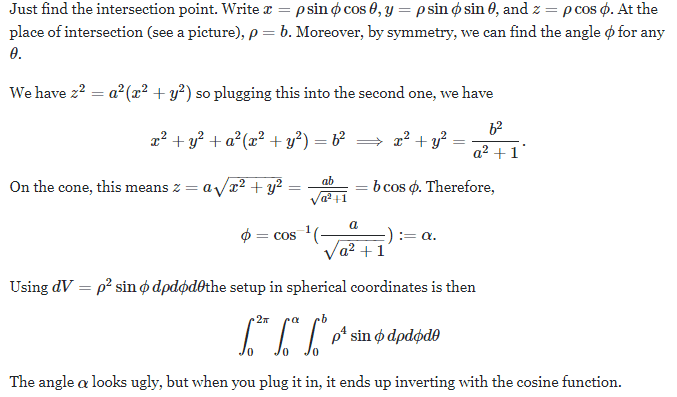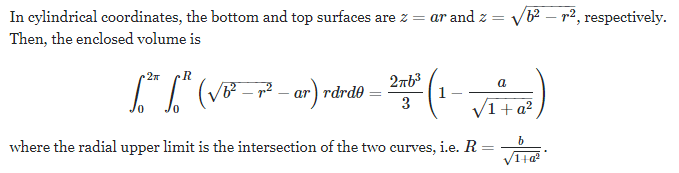# Which of the two answers is correct?

• I
• WMDhamnekar

#### WMDhamnekar

MHB
TL;DR Summary
Finding the volume above cone ##z= a\sqrt{x^2 + y^2}## and inside the sphere ## x^2 + y^2 + z^2 = b^2##Solution 1:The answer is ## \frac{2b^5\pi}{5} \times \left(1 -\frac{a}{\sqrt{1+a^2}}\right)##

Solution 2:I want to decide which answer is correct? Would you help me in this task?

•Delta2

I am with solution 2, easier to understand for me and I find it correct

After a closer look at Sol1. it computes not the volume V but the integral $$\iiint_V (x^2+y^2+z^2)dxdydz$$. The result of Sol1. would be the same as Sol2. if instead it would calculate $$\iiint_V 1 dxdydz=\int_0^{2\pi}\int_0^a\int_0^b \rho^2\sin\phi d\rho d\phi d\theta$$

•WMDhamnekar
After a closer look at Sol1. it computes not the volume V but the integral $$\iiint_V (x^2+y^2+z^2)dxdydz$$. The result of Sol1. would be the same as Sol2. if instead it would calculate $$\iiint_V 1 dxdydz=\int_0^{2\pi}\int_0^a\int_0^b \rho^2\sin\phi d\rho d\phi d\theta$$
Thanks for your scrutiny of both the solutions. But I want more confirmation from other elite members.

Thanks for your scrutiny of both the solutions. But I want more confirmation from other elite members.
Well ok, just to emphasize that the two solutions don't compute the same thing

We have a region V (as the region between the surface of a cone and a sphere).

Sol 1 computes ##\iiint_V (x^2+y^2+z^2)dxdydz##
Sol 2 computes ##\iiint_V 1 dxdydz##

Yes. Why do the solutions have different units ? Junk.

Yes. Why do the solutions have different units ? Junk.
Check post #5.

Yes.

•Delta2